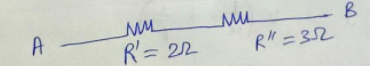Question

# Find the equivalent resistance between A and B.Medium
SolutionVerified by Toppr

## and are in parallel.So, their equivalent Resistance Also, and are in parallelSo, this equivalent Resistance Simplified circuit becomes :-and are in series.So, equivalent Resistance Therefore, equivalent Resistance between and is00